CATEGORIES:

# Decoder Networks

Large decoders are often required to perform decoding functions They useful, as universal modules, in implementing arbitrary switching functions. Since an n-input decoder has 2n outputs, it is not possible to implement a decoder for large n as a single module. Therefore, an n-input decoder with large n has to be implemented by a multimodule network. We discuss two approaches in designing these networks: coincident decoding and tree decoding.

Coincident decoding is introduced by the example in Figure 1.21, which implements an 8-input binary decoder using two 4-input binary decoders and 256 2-input AND gates. The 8-bit input vector is divided into two 4-bit subvectors, and each of these subvectors is used as input to one of the decoders. The outputs of the decoders correspond to minterms of the corresponding input variables. In this approach each output, which corresponds to an 8-variable minterm, is implemented by the AND function of the corresponding 4-variable products. For example, output z36 is the AND of the output w4, of one decoder and y2 of the other. This is so because 36 = 2 • 24 + 4.

The enable input of the decoder is connected to the enable of one of the decoder modules.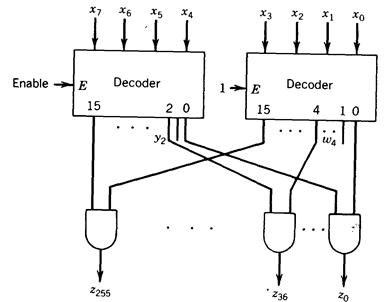Fig. 1.21 - Implementation of an 8-input decoder using two 4-input modules (coincident decoding).

In a generalization of this example, an n-input binary decoder is implemented by two (n/2)-input binary decoders W and Y and n2 AND gates, as indicated in Figure 1.22.Fig. 1.22 - Coincident decoder.

The input to the decoder W is xR = (xn/2-1 , . . . , x0) and the input to the de­coder Y is xL = (xn-1 , . . . , xn/2). The enable input is connected to the enable of one of the decoders (for example, Y). Therefore, the outputs of these decoders are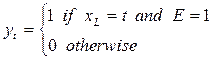The elements of the output vector z are obtained by the AND gates: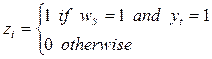The inputs to the AND gates are organized so that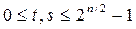Therefore, by substitution which corresponds to the description of an n-input decoder.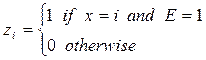A functional description that captures the structure of Figure 1.22.

z = (AND(y2n/2-1, w2n/2-1), … , AND(ys, wt), … , AND(y0, w0))

where

y = DEC(xL, E) and w = DEC(xR, 1)

If the number of inputs of a decoder module is less than n/2, then the coincident scheme can be applied by partitioning the input vector into more than two subvectors. If n=rk, where k is the number of inputs of a decoder module, then the scheme consists of r decoders and 2n r-input AND gates. For example, if n = 15 and k = 4, then three decoder modules and 4096 3-input AND gates are required as shown in Figure 1.23.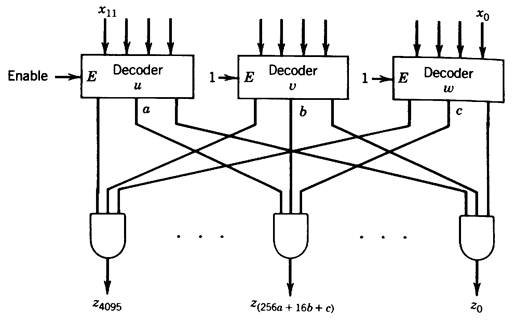Fig. 1.23 - A-12-input coincident decoder.

In this case,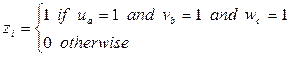for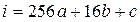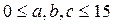whereand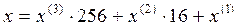.

Another scheme for the implementation of large decoders is a tree decoder. This approach is introduced by means of the 4-input decoder shown in Figure 1.24. The two-level tree has one decoder in the first level and four in the second level. The input vector x=(x3, x2, x1, x0) is partitioned into two subvectors, with (x3, x2) decoded in the first level and (x1, x0) in the second. The 16 outputs are partitioned into four groups of four outputs each; decoding of the subvector (x3, x2) enables one of the groups, and decoding of (x1, x0) produces the corresponding output in the enabled group.

In general, an n-input decoder can be implemented by a two-level tree with one n/2-input decoder in the first level and 2n/2 n/2-input decoders in the second level, as shown in Figure 1.25 input vector x is partitioned into two subvectors xR = (xn/2-1 , . . . , x0) and xL = (xn-1 , . . . , xn/2) such that x = xL · 2n/2 + xR.

The output of the first level is0 £ t £ 2n/2-1Fig. 1.24 - A 4-input tree decoder.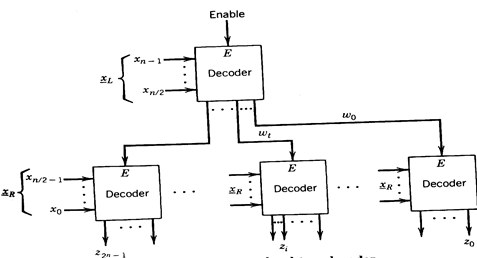Fig. 1.25 - A two-level tree decoder.

and the output of the second level,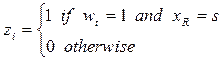Since i = t· 2n/2+s,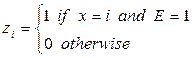and the network performs the decoding function on x.

A functional description of a two-level tree decoder is

z = (DEC(xR, w2n/2-1) , . . . . DEC(xR, wt) , . . . , DEC(xR, w0))

where w = DEC(xL, E).

This scheme can be generalized to a multilevel tree. If n=rk, at each level k input variables are introduced, producing a tree of r levels. The number of decoder mod­ules is

1 + 2k + 22k + . . . + 2(r - 1)k

which is equal to (2n - 1)/(2k - 1) since (r - 1)k=n - k.

We now compare the two approaches for implementing large decoders with re­spect to the number of modules, the delay, the fan-out, and the characteristics of the interconnections. To be specific we consider the case in which the implementa­tion is done using SSI and MSI chips. The chips to be used are a k-input decoder and a chip with m AND gates.

The coincident decoder requires n/k decoder chips and 2n/m AND chips (assuming that the AND gates have n/k inputs) and has a delay of one decoder-delay plus one AND-delay. The fan-out of the decoder outputs is 2n/k, and has (n/k) x 2n connections among the chips (n/k per AND gate). Note that the com­plexity of these connections depends on the way in which the AND gates are organized. For example, for the case in which k=n/2 a convenient organization is the two-dimensional array shown in Figure 1.22.

The tree decoder requires (2n-1)/(2k-1) decoder modules and has a delay of n/k decoder-delays. The fan-out of the external inputs is equal to the number of decoders in the corresponding level (maximum fan-out is 2n/k) and has (2n-1)/(2/c-1) internal connections (one per decoder module) but requires that the exter­nal inputs be connected to all decoders in that level.

We compare the two approaches in the following example.

A 6-input decoder is implemented using coincident and tree decoder schemes a illustrated in Figure 1.26.

The coincident scheme requires two 3-input decoders and 64 2-input AND gates. If implemented with standard SSI/MSI chips (four AND gates per chip), this scheme would require 18 chips. If the delay of a decoder and of an AND gate are both equal to d, the total delay is 2d. Each of the 16-decoder outputs is connected to 8 gates inputs for a total of 128 internal connections.

On the other hand, the tree decoder uses nine decoder chips, its delay is also equal 2d, and it has eight internal connections. In addition, the x2, x1, x0 must connected to eight decoder modules for a total of 24 connections.

Consequently, in this case, the tree decoder uses fewer chips and requires fewer connections.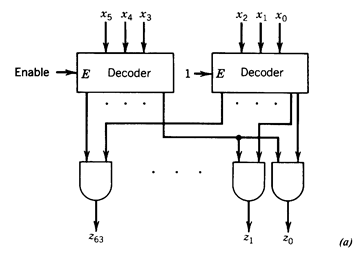Fig. 1.26 - Implementation of 6-input decoder. (a) Coincident decoder. (b) Tree decoder.

As seen in the example, in terms of the number of chips and interconnections, the tree-decoder scheme is better than the coincident one. Coincident decoding, on the other hand, allows a reduction of the complexity of connection between the generation of the decoding function and its use in other parts of the system. This is illustrated by the implementation of a 4-input ROM, which consists of a 4-input decoder and an array of cells that store the function table. If the decoder is a tree decoder, 24 = 16 lines go from the decoder to the array (Figure 1.27.a). On the other hand, if the decoder is a coincident decoder, then the AND gates can be part of the array of cells and only 2x24/2 = 8 lines are required (Figure 1.27.b). This difference is more significant for larger decoders.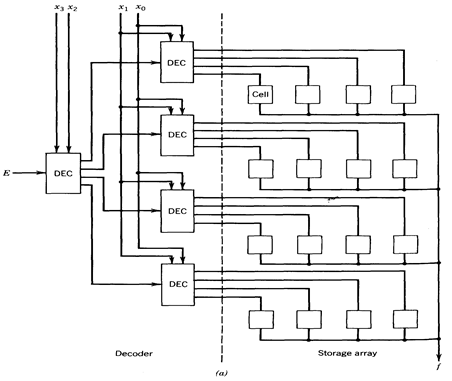.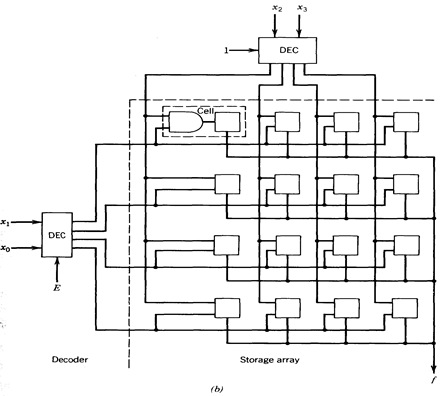Fig. 1.27 - (a) ROM with tree decoder (b) ROM with concident decoder.

Date: 2015-01-12; view: 3297

 <== previous page | next page ==> Binary Decoders | Coder units
doclecture.net - lectures - 2014-2023 year. Copyright infringement or personal data (0.014 sec.)